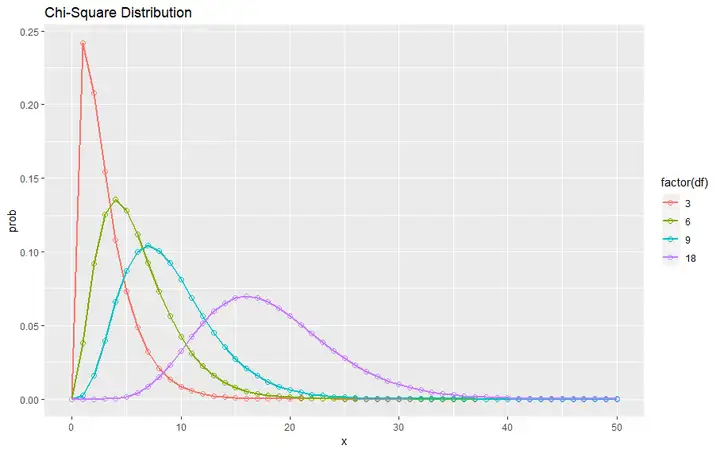# 卡方检验$\chi^2$

• $\chi^2$检验是非参数检验，就是我们根本不知道分类变量的分布。
• 比较理论频数和实际频数的吻合程度

• 假设每个面出现的概率为 $\frac{1}{6}$
• 设计实验：投骰子 $n$ 次，记录每个面出现的次数 $c_1 \cdots c_6$ ，用 $\frac{c_i}{n}(i=1, \ldots, 6)$ 求出每个面频率 $p_1 \ldots p_6$
• 用 $p_1 \ldots p_6$ 与 $\frac{1}{6}$ 比较，如果各个面的频率与 $\frac{1}{6}$ 相差不大，说明骰子是均匀的，如果每个面频 率与 $\frac{1}{6}$ 相差很大，说明骰子是不均匀的

# 什么是卡方$\chi^2$

$\chi^2$ 衡量观察值理论值之间的偏离程度

# 离散变量的关联性分析xtabs() 函数就能得到列联表，再用addmargins()函数就能得到带边际频数的列联表，用 addmargins(prop.table(...)) 函数就能得到带边际频率的列联表X-squared=129.29 跟我们算得一样

【注意】如果 $\chi^2$ 的值接近于 0 ，说明两者独立，几平没有什么关联性$p-$ value $<2.2 e-16$ 即 $p-$ value $<0.05$ ，拒绝原假设 $H_0$